## How to convert decimal degrees to degrees minutes seconds in Excel?

Sometimes, you may have a list of data shown as decimal degrees in a worksheet, and now you need to convert the decimal degrees to degrees, minutes and seconds formatting as shown as following screenshots, how can you get the conversation quickly in Excel?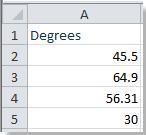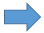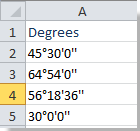Convert decimal degrees to degrees, minutes, seconds with VBA

Convert degrees, minutes, seconds to decimal degrees with VBA

####Convert decimal degrees to degrees, minutes, seconds with VBA

Please follow the steps below to convert decimal degrees to degrees, minutes, and seconds with VBA code.

1. Hold ALT button and press F11 on the keyboard to open a Microsoft Visual Basic for Application window.

2. Click Insert > Module, and copy the VBA into the module.

VBA: Convert decimal degree to degree, minutes and seconds

``````Sub ConvertDegree()
'Update 20130815
Dim Rng As Range
Dim WorkRng As Range
On Error Resume Next
xTitleId = "KutoolsforExcel"
Set WorkRng = Application.Selection
Set WorkRng = Application.InputBox("Range", xTitleId, WorkRng.Address, Type:=8)
For Each Rng In WorkRng
num1 = Rng.Value
num2 = (num1 - Int(num1)) * 60
num3 = Format((num2 - Int(num2)) * 60, "00")
Rng.Value = Int(num1) & "°" & Int(num2) & "'" & Int(num3) & "''"
Next
End Sub
``````

3. Click Run button or press F5 to run the VBA.

4. A dialog displayed on the screen, and you can select the cells you want to the convert. See screenshot: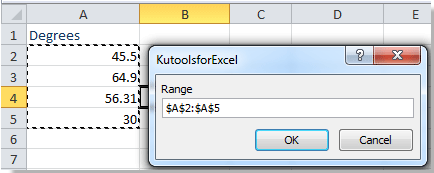5. Click OK, then the selected data is converted to degree, minutes and seconds. See screenshot:Tip: Using the above VBA code will lost your original data, so you’d better copy the data before running the code.

####Convert degrees, minutes, seconds to decimal degrees with VBA

Sometimes, you may want to convert the data in degrees/minutes/seconds formatting to decimal degrees, the following VBA code can help you quickly get it done.

1. Hold ALT button and press F11 on the keyboard to open a Microsoft Visual Basic for Application window.

2. Click Insert > Module, and copy the VBA into the module.

VBA: Convert degree, minutes and seconds to decimal degree

``````Function ConvertDecimal(pInput As String) As Double
'Updateby20140227
Dim xDeg As Double
Dim xMin As Double
Dim xSec As Double
xDeg = Val(Left(pInput, InStr(1, pInput, "°") - 1))
xMin = Val(Mid(pInput, InStr(1, pInput, "°") + 2, _
InStr(1, pInput, "'") - InStr(1, pInput, _
"°") - 2)) / 60
xSec = Val(Mid(pInput, InStr(1, pInput, "'") + _
2, Len(pInput) - InStr(1, pInput, "'") - 2)) _
/ 3600
ConvertDecimal = xDeg + xMin + xSec
End Function
``````

3. Save the code and close the window, select a blank cell, for instance, the Cell A1, enter this formula =ConvertDecimal("10° 27' 36""") ("10° 27' 36""" stands the degree you want to convert to decimal degree, you can change it as you need), then click Enter button. See screenshots: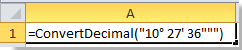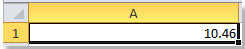#### Relative articles

No ratings yet. Be the first to rate!
This comment was minimized by the moderator on the site
WRONG calculation results... 29°30'13" 34°55'4" ---> 29.00083333 34.08333333
This comment was minimized by the moderator on the site
The code has a assumes there is a space after ° and '. Change the 2's to 1's in the code to solve it.
Function ConvertDecimal(pInput As String) As Double
'Updateby20140227
Dim xDeg As Double
Dim xMin As Double
Dim xSec As Double
xDeg = Val(Left(pInput, InStr(1, pInput, "°") - 1))
xMin = Val(Mid(pInput, InStr(1, pInput, "°") + 1, _
InStr(1, pInput, "'") - InStr(1, pInput, _
"°") - 1)) / 60
xSec = Val(Mid(pInput, InStr(1, pInput, "'") + _
1, Len(pInput) - InStr(1, pInput, "'") - 1)) _
/ 3600
ConvertDecimal = xDeg + xMin + xSec
End Function
This comment was minimized by the moderator on the site
Works like a champ! Thanks for sharing!!!
This comment was minimized by the moderator on the site
Check your results. this code is wrong.
This comment was minimized by the moderator on the site
please i want you help and write a computer program on paper to convert decimal of degree to degree minute and second. 317.5986740026 from ahans.
This comment was minimized by the moderator on the site
step 1, Multiply numbers after decimal with 60 (0.5986740026*60)minutes=35.92044015 mean 35 mints
spet 2, multiply the decimal after minute ie 0.9204401*60=55.2264
so 317 degree 35 minuts 55 sec
This comment was minimized by the moderator on the site
Not sure whats wrong with the code in the post from Ernie, but this is what I use to convert decimal degrees to DMS degrees in the spread sheet without using VB. The reference to the cell E33 is the cell containing the decimal degrees value. =CONCAT(FIXED(INT(B32), 0) , "°", FIXED(((B32 - INT(B32)) * 60), 0), "'", FIXED(((B32 - INT(B32) - INT(B32 - INT(B32))) * 3600), 0 ,TRUE), """) Good luck.
This comment was minimized by the moderator on the site
HI CORNY, NOT WORK FOR ME, EXCEL DO NOT ACCEPT THE FUNCTION..... WHATS WRONG? CAN YOU HELP ME?
This comment was minimized by the moderator on the site
Hello, I used the scrip to convert decimal to DMS however the code is wrong somewhere as it turned out 37.856908,-120.912469 to 37D 51M 25S, -121D 5M 15S This is way off.... Anyone have a fix for this?
This comment was minimized by the moderator on the site
works great but how would i adapt the code to get more numbers for seconds?
This comment was minimized by the moderator on the site
I want to plot the positions on a map and we not worry about N, S, E or W. I'm sure these could be added in with "if" statements. I have just used a very simple line of functions within the Excel spreadsheet. I want to go from Degrees (Decimal) to Degrees, Seconds (Decimal) Changing the negative values to positives as I know which hemispheres I am in. For me, cell E4 contained the target Lat or Long (Decimal). This is a bit easier than putting in some VBA code and can be enlarged to do DD,MM,SS =CONCATENATE(TRUNC(ABS(E4)),"°",FIXED((ABS(E4)-TRUNC(ABS(E4)))*60,2),"'")
This comment was minimized by the moderator on the site
Found this code very helpful. Great stuff!
This comment was minimized by the moderator on the site
The most important thing to realize is that you cannot simply use superscript and small letter o for the degree symbol - this may be obvious to some, but not to me. I tried the superscript letter o and it did not work, I deleted the superscript letter o, and used Alt 0176 for the actual degree symbol and it worked!! I am confused as to why after the seconds there are extra sets of double quotes? 36""") whereas in the subsequent comment if one simply uses =ConvertDecimal(A1) only a single set of quotes are used for seconds. Odd we can use single and double quotes and the thing works for minutes and seconds but superscript letter o does not? To check 27/60 = .45 smf 36/3600 = .01 add the two = .46 and add that to the degrees = 10.46 degrees. Hope this helps others? Mort
There are no comments posted here yet